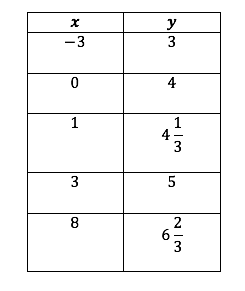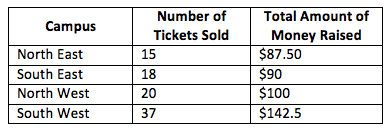Match Fishtank is now Fishtank Learning!

# Linear Equations, Inequalities and Systems

## Objective

Identify the solutions and features of a linear equation and when two linear equations have the same solutions.

## Common Core Standards

### Core Standards

?

• A.REI.D.10 — Understand that the graph of an equation in two variables is the set of all its solutions plotted in the coordinate plane, often forming a curve (which could be a line).

• A.SSE.B.3 — Choose and produce an equivalent form of an expression to reveal and explain properties of the quantity represented by the expression. Modeling is best interpreted not as a collection of isolated topics but in relation to other standards. Making mathematical models is a Standard for Mathematical Practice, and specific modeling standards appear throughout the high school standards indicated by a star symbol (★). The star symbol sometimes appears on the heading for a group of standards; in that case, it should be understood to apply to all standards in that group.

?

• 8.EE.C.7

• 8.F.A.2

• 8.F.B.4

## Criteria for Success

?

1. Identify that the solutions to a line are only those that are on the graph of the line or can be substituted into a linear equation to produce a true statement.
2. Show algebraically that a point is either on the graph of a line or not on the graph of a line.
3. Manipulate a linear equation to show that it has the same solutions as an original equation.

## Anchor Problems

?

### Problem 1

Name at least four points that are solutions to the linear equation ${4x-y=10}$.

### Problem 2

The following two equations have identical solutions.

${y=\frac{1}{3}x+4}$

${-12=x-3y}$

Explain how you know that:

• The two equations have identical solutions.
• The following table of values represents a set of solutions to both equations.## Problem Set

?

The following resources include problems and activities aligned to the objective of the lesson that can be used to create your own problem set.

• Include problems that provide the equation and a set of values. Ask students to identify the coordinate points that are solutions to the equation.

?

Several campuses are holding fundraisers and tallied the amount of tickets they sold, the amount of money they raised, and any one-time donations.

The prediction for how each campus would price the tickets, plus one-time donations was:  ${y=2.5x + 50}$

Part A
Which campuses, based on the amount of tickets sold and the total amount raised, appear to have followed the model prediction?Part B
Central East and Central West forgot to send in their total amounts of money raised. The director used the prediction equation and his best guess of a sale of 25 tickets and 42 tickets, respectively. How much money did the director predict Central East and Central West raised?